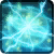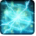# Sphère d'énergieConditions

## Related effects, buffs and debuffs

Please click on an effect below to view its details.

• [hidden] [28s]Effect 4167333752865025

 Slot: Buff Duration: 28s Tick rate: 2.6s # occurrences: 0
• On Apply

Perform the following actions:

• Play appearance epp . operation . iokath . boss . izax . energy_drone . p5_energy_ball, dependent on calling effect
• When effect ticks the 1th time

Perform the following actions:

• Add effect #2 to TARGET from TARGET
• [hidden] [23s]Effect 4167333752865041

 Slot: Buff Duration: 23s Tick rate: does not tick # occurrences: 0 Conditions: Can only by called by other effects
• On Apply

Perform the following actions:

• Unknown (183)
- Unknown (303) = (bool) true
- Unknown (306) = (bool) true
- Unknown (428) = (bool) false
- Unknown (430) = (bool) false
- Unknown (310) = (string) aoePositionOptionType_Entity
- Unknown (311) = (string) aoeTarget_Caster
- Unknown (313) = (string) aoeScalarType_LiteralValue
- Unknown (317) = (string) aoeScalarType_LiteralValue
- Unknown (321) = (string) aoeScalarType_LiteralValue
- Unknown (325) = (string) aoeOrientationOptionType_WorldCoordinates
- Unknown (344) = (string) aoeSphere
- Unknown (349) = (string) aoeTargetCondition_IsEnemy[aoeParameter_Enemyof:aoeConditionTarget_Caster]
- Unknown (353) = (string) aoeScalarType_LiteralValue
- Unknown (354) = (string) aoeScalarType_LiteralValue
- Unknown (355) = (string) aoeScalarType_LiteralValue
- Unknown (367) = (string) aoeScalarType_LiteralValue
- Unknown (384) = (string)
- Unknown (386) = (string) aoeAbilityCaster_Caster
- Unknown (387) = (string) aoeAbilityTarget_Target
- Unknown (407) = (string) aoeMovement_DirectedMotion
- Unknown (414) = (string) aoeScalarType_LiteralValue
- Unknown (418) = (string) aoeScalarType_LiteralValue
- Unknown (422) = (string) aoeScalarType_LiteralValue
- Unknown (429) = (string) aoeMovement_ContinueMoving
- Unknown (431) = (string) aoeRotation_None
- Ability Spec = (int) 16140971409235029938
- Unknown (307) = (int) 1
- Unknown (308) = (int) 46
- Tick Rate = (float) 0.5
- Unknown (304) = (float) 0.5
- Unknown (305) = (float) 23
- Unknown (314) = (float) 0
- Unknown (318) = (float) 0
- Unknown (322) = (float) 0
- Unknown (362) = (float) 0
- Unknown (363) = (float) 0
- Unknown (364) = (float) 0
- Unknown (368) = (float) 0.45
- Unknown (390) = (float) 0
- Unknown (398) = (float) 0.5
- Unknown (417) = (float) 1
- Unknown (421) = (float) 0
- Unknown (425) = (float) 0
- Unknown (426) = (float) 0.335
- Unknown (427) = (float) 1000